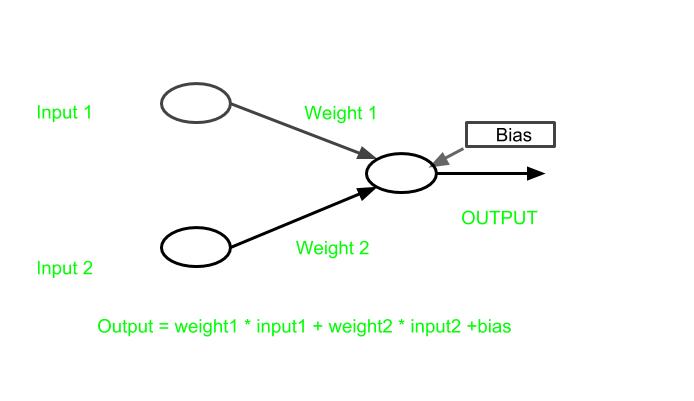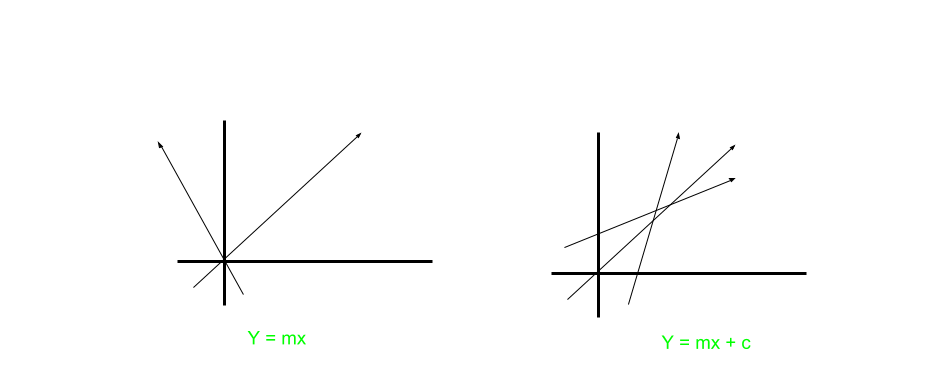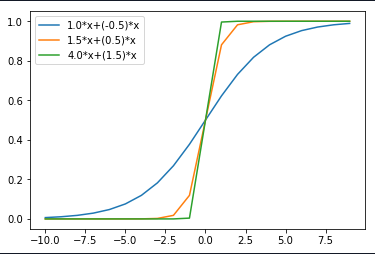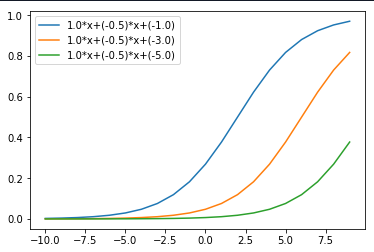# Effect of Bias in Neural Network

Neural Network is conceptually based on actual neuron of brain. Neurons are the basic units of a large neural network. A single neuron passes single forward based on input provided.

In Neural network, some inputs are provided to an artificial neuron, and with each input a weight is associated. Weight increases the steepness of activation function. This means weight decide how fast the activation function will trigger whereas bias is used to delay the triggering of the activation function.

For a typical neuron, if the inputs are x1, x2, and x3, then the synaptic weights to be applied to them are denoted as w1, w2, and w3.

Output is

## `y = f(x) = Σxiwi`

where i is 1 to the number of inputs.

The weight shows the effectiveness of a particular input. More the weight of input, more it will have impact on network.

On the other hand Bias is like the intercept added in a linear equation. It is an additional parameter in the Neural Network which is used to adjust the output along with the weighted sum of the inputs to the neuron. Therefore Bias is a constant which helps the model in a way that it can fit best for the given data.

The processing done by a neuron is thus denoted as :

## `output = sum (weights * inputs) + bias`### Need of bias

In above figure

`y = mx+c`

where

`m  =  weight`

and

`c  =  bias`

Now, Suppose if c was absent, then the graph will be formed like this:Due to absence of bias, model will train over point passing through origin only, which is not in accordance with real-world scenario. Also with the introduction of bias, the model will become more flexible.

For Example:
Suppose an activation function act() which get triggered on some input greater than 0.
Now,

`input1 = 1`
`weight1 = 2`
`input2 = 2`
`weight2 = 2`

so

`output  =  input1*weight1 + input2*weight2`
`output  =  6`

let

`suppose act(output)  =  1`

Now a bias is introduced in output as

`bias  =  -6`

the output become 0.

`act(0)  =  0`

so activation function will not trigger.

### Change in weight

Here in graph, as it can be seen that when:

• weight WI
` changed from  1.0 to 4.0 `
• weight W2
` changed from  -0.5 to 1.5 `

On increasing the weight the steepness is increasing.

Therefore it can be inferred that

`More the weight earlier activation function will trigger.`### Change in bias

Here in graph below, when

`Bias changed from  -1.0 to -5.0`

The change in bias is increasing the value of triggering activation function.Therefore it can be inferred that from above graph that,

`bias helps in controlling the value at which activation function will trigger.`
My Personal Notes arrow_drop_upCheck out this Author's contributed articles.

If you like GeeksforGeeks and would like to contribute, you can also write an article using contribute.geeksforgeeks.org or mail your article to contribute@geeksforgeeks.org. See your article appearing on the GeeksforGeeks main page and help other Geeks.

Please Improve this article if you find anything incorrect by clicking on the "Improve Article" button below.

Article Tags :
Practice Tags :

3

Please write to us at contribute@geeksforgeeks.org to report any issue with the above content.